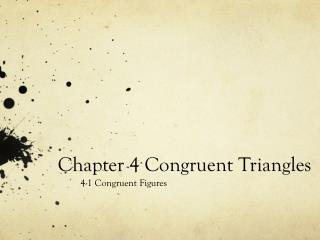DownloadDownload PresentationChapter 4 Congruent Triangles

# Chapter 4 Congruent Triangles

Download Presentation## Chapter 4 Congruent Triangles

- - - - - - - - - - - - - - - - - - - - - - - - - - - E N D - - - - - - - - - - - - - - - - - - - - - - - - - - -
##### Presentation Transcript

1. Chapter 4 Congruent Triangles 4-1 Congruent Figures

2. Objectives • Students will be able to: • Recognize congruent figures and their corresponding parts • Define and apply the third angles theorem • Use congruent parts to prove triangles congruent

3. Essential Understanding • You can determine whether two figures are congruent by comparing their corresponding parts.

4. Congruent Figures • What do you know about congruent figures? • Does the orientation of congruent figures matter? • No, the congruent figures can be slid, flipped, or turned and they will still be congruent

5. Congruent Figures • Congruent Polygons: • Have congruent corresponding parts (equal sides and angles) • When naming congruent figures, you must list corresponding parts (angles/vertices) in the same order

6. Corresponding Parts • If figure HIJK ≅ LMNO, what are the congruent corresponding parts? • If it helps, draw a picture • Then name the corresponding segments • HI ≅ LM IJ ≅ MN JK ≅ NO KH ≅ OL • Then name the corresponding angles • <H ≅ <L <I ≅ <M <J ≅ <N <K ≅ <O • If ΔABC ≅ ΔXYZ, what are the congruent corresponding parts?

7. Congruent Parts • Suppose ΔABC ≅ ΔFED, <B = 30 and <D = 75, what is the measure of <A? • Draw a picture of the situation • Suppose ΔWYS ≅ ΔMKV. If <W = 62 and <Y = 35, what is the measure of <V? How do you know?

8. Third Angles Theorem • If you know two angles of a triangle are congruent, then the third angles will be congruent.

9. Are the triangles congruent? • Write a two column proof • To prove two triangle congruent you need to get all the corresponding angles and segments to be congruent. This allows you to use the Def. of Congruent Triangles reason as your final statement • Statements | Reasons . • 1. AB ≅ AD, BC ≅ DC, 1. Given<B ≅ <D • 2. AC ≅ AC 2. Reflexive Property of ≅ • 3.<BCA ≅ <DCA 3. All right angles are congruent • 4. <BAC ≅ <DAC 4. Third <s Thm. • 5. ΔABC ≅ ΔADC 5. Def of Congruent Triangles

10. Are the Triangles Congruent? • No because we cannot show that all the corresponding parts are congruent.

11. Proving Triangles Congruent • Given: <A ≅ <D, AE ≅ DC, EB ≅ CB, BA ≅ BD • Prove: ΔAEB ≅ΔDCB

12. Homework • Pg. 222 -223 • 10 – 19, 30 – 32, 44 • 14 problems